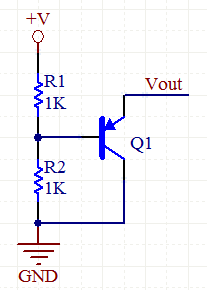# Variable Zener Circuit

A zener diode acts like a normal diode, blocking current when it is reverse biased until a threshold voltage is surpassed, then it conducts Zener diodes are often used in voltage regulators.

The circuit below simulates a zener diode and is programmable with a resistor divider network. The zener voltage is equal to:

Vz= (R2*V)/(R1+R2)+Vbe

Where Vbe is the voltage drop from the emit to the base of the transistor, and which is normally about 0.6VVariable Zener Schematic